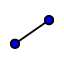# Lab13 Welcome to the Matrix

## 1) Geometry

Begin this lab by cutting out the outline of your geometric shape. If you were not given a paper, print one here. Fold the edge flaps and tape them together.

## 2) Computer Science

Ever wonder how to take that 3-D object in your hand and put it into a computer? Use your geometric figure to follow along with the Computerphiles video. Jot down the connection between the various matrix operations and the effect on the graph.

## 3) Math

Explore the GeoGebra applets below. Homer Simpson would be encoded into a computer using a massive matrix to plot each point on his face as well as a corresponding color code. To explore the effect of multiplying by the transformation matrix, change some of the numbers in the matrix and see how Homer changes. Can you make his image reflect over the x-axis, y-axis, rotate, dilate?

## 4) Create

Create a new 3-D graphics page using GeoGebra. Plot your pyramid in the x,y,z coordinate plane. To do this, the five vertices in (x,y,z) form and then connect them with usingthe line segment tool OR typing the command Segment[<Point>,<Point>]. Paste a screenshot in your lab (from a good angle).